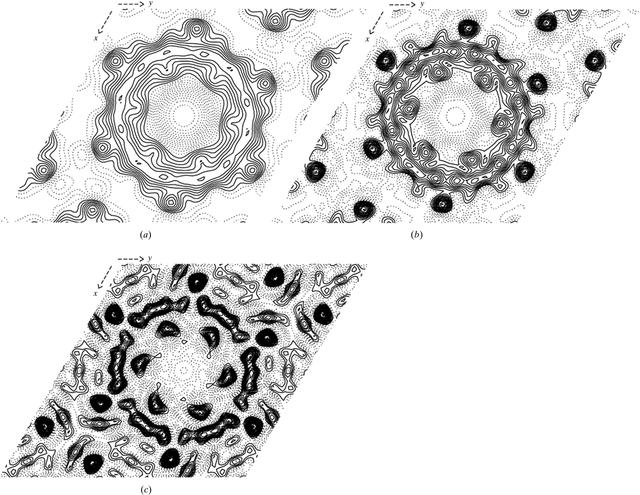disable zoom     view article Figure 3 Effect of inverse B factors on projection density maps. Inverse B factors are frequently used in electron crystallography to overcome the resolution-dependent fall-off of image-derived amplitudes in cases where no electron diffraction data are available. Three projection density maps of a single truncated gap-junction channel are shown. Each map was calculated to a nominal resolution of 6 Å. Inverse B factors of B = 0 and −350 Å2 were used in (a) and (b), respectively. The map shown in (c) was calculated after setting all amplitudes to unity and using (FOM*cosαav) (see Fig. 2b) as a weight in the Fourier inversion. All three maps are contoured in increments of 0.25σ. Solid lines are drawn for contour levels above the mean. Density maps were generated using the CCP4 program package (Collaborative Computational Project, Number 4, 1994). The `sharpening-factor' (sf) is obtained as sf = exp[−B/(4d*d)], where B is the B factor and d is the resolution in Å. For instance, B = −350 Å2 increases the amplitude of a reflection at 6 Å by ∼11-fold, provided that its FOM is 1. For FOM < 1, the boost will be reduced to (FOM*sf). Similarly, applying (FOM*cosαav) (see Fig. 2b) as a weight in plane groups with twofold constraint ensures that the full amount of sharpening is applied only to the most reliable reflections. In this case, even amplitudes as incorrect as those used for the map shown in (c) will result in a `density distribution' that still describes the main features of the projected structure correctly. In case of the gap-junction channel, these features are two groups of six roughly circular and rotationally staggered densities that are separated by a continuous band of density.BIOLOGICALCRYSTALLOGRAPHY
ISSN: 1399-0047
Volume 56| Part 10| October 2000| Pages 1259-1269